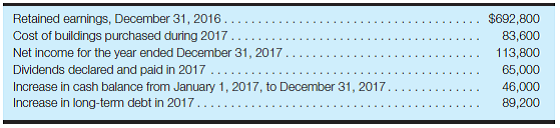# Accounting

## Quiz 8 : Accounting for and Presentation of Stockholders EquityStudy FlashcardsLooking for Accounting Homework Help?

## Quiz 8 :Accounting for and Presentation of Stockholders Equity

Question TypePreferred stock-calculate dividend amounts Rosie, Inc., did not pay dividends on its $4.50,$50 par value, cumulative preferred stock during 2015 or 2016, but had met its preferred dividend requirement in all prior years. Since 2011, 42,000 shares of this stock have been outstanding. Rosie, Inc., has been profitable in 2017 and is considering a cash dividend on its common stock that would be payable in December 2017. Required: Calculate the amount of dividends that would have to be paid on the preferred stock before a cash dividend could be paid to the common stockholders.
Free
Essay

Preferred stock-compute dividend amounts
Preferred stock: Preferred stock usually has a dividend that should be paid out earlier than dividends to common or equity stockholders.
The Preferred dividends would have to be paid for 2015 and 2016 also with current year dividend (2017). Thus, the computation of total dividend paid on the preferred stock is as follows:
Step 1: To calculate Annual dividend;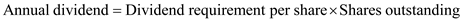Substitute: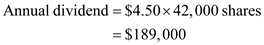Step 2: To calculate total dividend paid in the current year; Arrears of dividends for last 2 years is also yet to be paid in the current year, thus the total dividends to be paid is: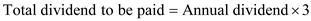Substitute: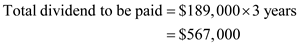Therefore, the total of dividends to be paid in the current year is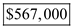.

Tags
Choose question tagPreferred stock-calculate dividend amounts Laura Marty, Ltd., did not pay dividends on its 9.5%, $100 par value cumulative preferred stock during 2015 or 2016. Since 2009, 175,000 shares of this stock have been outstanding. Laura Marty, Ltd., has been profitable in 2017 and is considering a cash dividend on its common stock that would be payable in December 2017. Required: Calculate the amount of dividends that would have to be paid on the preferred stock before a cash dividend could be paid to the common stockholders. Free Essay Answer: Answer: Cumulative dividends are one of the preferences given to preferred stockholders. It mandates that any missed dividends to preferred stockholders must be paid before any dividends are paid on common stock. Company S J did not pay dividends on their preferred stock in 2015 or 2016. Before they can issue a cash dividend in 2017 to their common stockholders, they are required to pay cumulative dividends for 2015, 2016, and 2017. In order to calculate the annual dividend amount owed to preferred stockholders, multiply the stated dividend rate by the par value per share of the preferred stock by the total number of shares outstanding.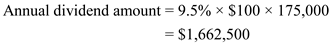The total amount of dividends paid for 2015, 2016 and 2017 is calculated as the annual dividend amount multiplied by the number of years.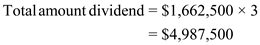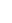Therefore, the answer is$4,987,500.

Tags
Choose question tagReview exercise-calculate retained earnings From the following data, calculate the Retained Earnings balance as of December 31, 2016: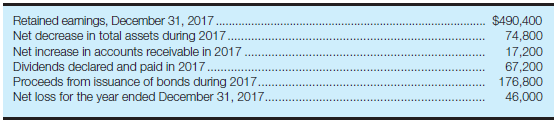Free
Essay

Retained earnings are the balance of earnings that are earned by the company over a period of years after paying for dividends. In general, net income earned by the company (after adjusting for interest expense and income tax) is transferred to retained earnings account and thereafter dividends declared and paid are deducted from that amount of retained earnings balance. Retained earnings are part of stockholder's equity.
Retained earnings reflect the cumulative earnings of the company that have been retained for use in the business rather than disbursed to the stockholders as dividends. Virtually the only factors affecting retained earnings are net income or loss reported on the income statement, and dividends. It means the retained earnings will be affected only for the net income or net loss and dividends declared if any. Except these, no other transaction will affect the retained earnings of the company.
In the present case, the only transactions that affect the retained earnings of the company are net loss during the year and dividends declared and paid. Other transactions like decrease in total assets, increase in accounts receivable and proceeds from the issuance of bonds will not affect.
Calculate the retained earnings balance as of December 31, 2016:
Since we have the ending balance of retained earnings for the year 2017, we need to add the net loss for the year 2017 and dividends declared and paid during the year for calculating the opening balance of retained earnings for the year 2017 (Ending balance of December 2016).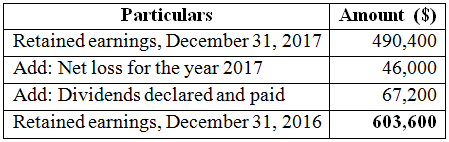The balance of retained earnings as of December 31, 2016 is $603,600. Tags Choose question tagDividend dates-market price effects O'Garro, Inc., has paid a regular quarterly cash dividend of$0.70 per share for several years. The common stock is publicly traded. On February 21 of the current year, O'Garro's board of directors declared the regular first-quarter dividend of $0.70 per share payable on March 30 to stockholders of record on March 15. Required: As a result of this dividend action, state what you would expect to happen to the market price of the common stock of O'Garro, Inc., on each of the following dates. Explain your answers. a. February 21. b. March 13. c. March 15. d. March 30. Essay Answer: Tags Choose question tagPreferred stock-calculate dividend amounts Calculate the cash dividends required to be paid for each of the following preferred stock issues: Required: a. The semiannual dividend on 9% cumulative preferred,$50 par value, 30,000 shares authorized, issued, and outstanding. b. The annual dividend on $6.20 cumulative preferred, 500,000 shares authorized, 160,000 shares issued, 127,400 shares outstanding. Last year's dividend has not been paid. c. The quarterly dividend on 6.5% cumulative preferred,$100 stated value, $103 liquidating value, 200,000 shares authorized, 168,000 shares issued and outstanding. No dividends are in arrears. Essay Answer: Tags Choose question tagCommon stock-issuance and dividend transactions Altuve Co. was incorporated on January 1, 2016, at which time 250,000 shares of$1 par value common stock were authorized, and 140,000 of these shares were issued for $12 per share. Net income for the year ended December 31, 2016, was$1,200,000. Altuve Co.'s board of directors declared dividends of $2 per share of common stock on December 31, 2016, payable on February 7, 2017. Required: Use the horizontal model (or write the entry) to show the effects of a. The issuance of common stock on January 1, 2016. b. The declaration of dividends on December 31, 2016. c. The payment of dividends on February 7, 2017. Essay Answer: Tags Choose question tagReview exercise-calculate net income At the beginning of the current fiscal year, the balance sheet of Hughey, Inc., showed stockholders' equity of$260,000. During the year, liabilities increased by $11,000 to$116,000; paid-in capital increased by $20,000 to$90,000; and assets increased by $130,000. Dividends declared and paid during the year were$28,000. Required: Calculate net income or loss for the year. (Hint: Set up the accounting equation for beginning balances, changes during the year, and ending balances; then solve for missing amounts.)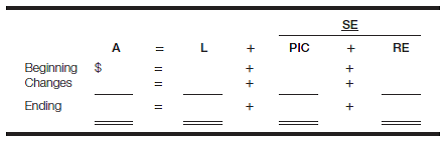Essay
Tags
Choose question tagCash dividends versus stock dividends Under what circumstances would you (as an investor) prefer to receive cash dividends rather than stock dividends? Under what circumstances would you prefer stock dividends to cash dividends?
Essay
Tags
Choose question tagInterpret dividend information from an annual report Refer to the Campbell Soup Company annual report in the appendix. From the table of quarterly financial information and the Selected Financial Data (Five-Year Review-Consolidated), find the information relating to cash dividends on common stock. Required: a. How frequently are cash dividends paid? b. What has been the pattern of the cash dividend amount per share relative to the pattern of earnings per share? c. Calculate the rate of change in the annual dividend per share for each of the years from 2011 through 2014.
Essay
Tags
Choose question tagRequirements for declaring dividends Anglin, Inc., expects to incur a loss for the current year. The chairperson of the board of directors wants to have a cash dividend so that the company's record of having paid a dividend during every year of its existence will continue. What factors will determine whether the board can declare a dividend?
Essay
Tags
Choose question tagCommon stock-calculate issue price and dividend amount The balance sheet caption for common stock is the following: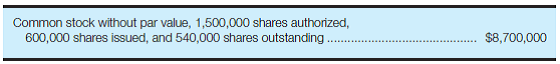Required: a. Calculate the average price at which the shares were issued. b. If these shares had been assigned a stated value of $1 each, show how the caption here would be different. c. If a cash dividend of$1.20 per share were declared, calculate the total amount of cash that would be paid to stockholders. d. What accounts for the difference between issued shares and outstanding shares?
Essay
Tags
Choose question tagReview exercise-calculate net income At the beginning of the current fiscal year, the balance sheet of Cummings Co. showed liabilities of $876,000. During the year, liabilities decreased by$144,000; assets increased by $308,000; and paid-in capital increased by$40,000 to $760,000. Dividends declared and paid during the year were$248,000. At the end of the year, stockholders' equity totaled $1,516,000. Required: Calculate net income or loss for the year using the same format as shown in Exercise 8.5. Reference of Exercise 8.5 Review exercise-calculate net income At the beginning of the current fiscal year, the balance sheet of Hughey, Inc., showed stockholders' equity of$260,000. During the year, liabilities increased by $11,000 to$116,000; paid-in capital increased by $20,000 to$90,000; and assets increased by $130,000. Dividends declared and paid during the year were$28,000. Required: Calculate net income or loss for the year. (Hint: Set up the accounting equation for beginning balances, changes during the year, and ending balances; then solve for missing amounts.)Essay
Tags
Choose question tagCalculate stock dividend shares and cash dividend amounts Assume that you own 6,000 shares of Briant, Inc.'s common stock and that you currently receive cash dividends of $2.52 per share per year. Required: a. If Briant, Inc., declared a 5% stock dividend, how many shares of common stock would you receive as a dividend? b. Calculate the cash dividend per share amount to be paid after the stock dividend that would result in the same total cash dividend (as was received before the stock dividend). c. If the cash dividend remained at$2.52 per share after the stock dividend, what per share cash dividend amount without a stock dividend would have accomplished the same total cash dividend? d. Why might a company consider having a dividend policy of paying a $0.30 per share cash dividend every year and also issuing a 5% stock dividend every year? Essay Answer: Tags Choose question tagEx-dividend date-market price effect Find a list of common stock ex-dividend date data. You can go, via Google, to wsj.com. Under the Markets tab, select Market Data; then click on the arrow next to U.S. Stocks to open the pull down menu and select Dividends under the Stocks and Trading Statistics category. Scroll down to the Dividend Declarations Table, which includes the relevant ex-dividend dates. Select several stocks, preferably of large and well-known companies. Go to a stock listing on the ex-dividend date and determine what happened to the market price of a share of stock on that date. Does this price action make sense? Explain your answer. Essay Answer: Tags Choose question tagCommon stock balance sheet disclosure The balance sheet caption for common stock is the following: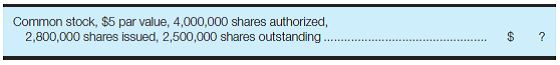Required: a. Calculate the dollar amount that will be presented opposite this caption. b. Calculate the total amount of a cash dividend of$0.30 per share. c. What accounts for the difference between issued shares and outstanding shares?
Essay
Tags
Choose question tagEffects of a stock split Assume that you own 3,600 shares of $10 par value common stock and the company has a 4-for-1 stock split when the market price per share is$68. Required: a. How many shares of common stock will you own after the stock split? b. What will probably happen to the market price per share of the stock? c. What will probably happen to the par value per share of the stock?
Essay
Tags
Choose question tagPreferred stock-calculate dividend amounts Dedrick, Inc., did not pay dividends in 2015 or 2016, even though 70,000 shares of its 8.5%, $50 par value cumulative preferred stock were outstanding during those years. The company has 500,000 shares of$2 par value common stock outstanding. Required: a. Calculate the annual dividend per share obligation on the preferred stock. b. Calculate the amount that would be received by an investor who has owned 1,400 shares of preferred stock and 16,000 shares of common stock since 2014 if a $0.60 per share dividend on the common stock is paid at the end of 2017. Essay Answer: Tags Choose question tagTreasury stock transactions On April 10, 2016, Amelia, Inc., purchased 800 shares of its own common stock in the market for$27 per share. On September 28, 2016, the company sold 500 of these shares in the open market at a price of $31 per share. Required: Use the horizontal model (or write the entry) to show the effects on Amelia, Inc.'s financial statements of a. The purchase of the treasury stock on April 10, 2016. b. The sale of the treasury stock on September 28, 2016. Essay Answer: Tags Choose question tagPreferred stock-calculate dividend amounts Calculate the annual cash dividends required to be paid for each of the following preferred stock issues: Required: a.$4.75 cumulative preferred, no par value; 400,000 shares authorized, 325,000 shares issued. (The treasury stock caption of the stockholders' equity section of the balance sheet indicates that 40,600 shares of this preferred stock issue are owned by the company.)b. 5%, $50 par value preferred, 200,000 shares authorized, 172,000 shares issued, and 68,500 shares outstanding. c. 7.4% cumulative preferred,$100 stated value, \$104 liquidating value; 80,000 shares authorized, 63,200 shares issued, 57,600 shares outstanding.
EssayReview exercise-calculate retained earnings From the following data, calculate the Retained Earnings balance as of December 31, 2017: